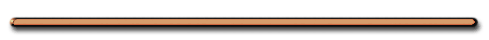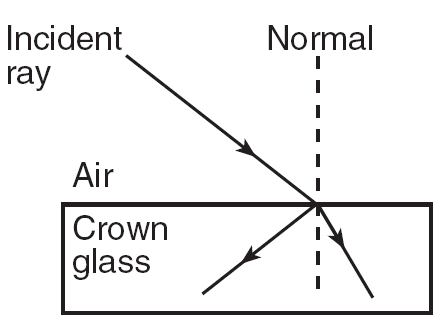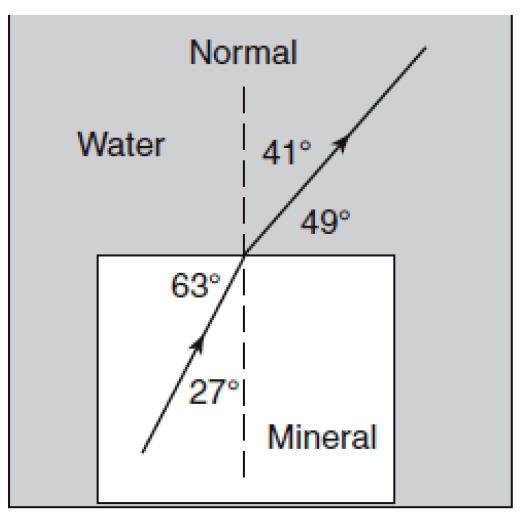HomeA wave entering a slower medium

[ Flash ]

Is this refraction? Angle of incidence?

No. The wave is changing speed but not bending.

a) Which wave characteristic changes? stays the same?

b) How many times faster is the blue medium than yellow medium?

[ Flash ]

a) wavelength decreases in blue medium

b) frequency is the same

c) Blue medium is twice as fastb. n and V

 n1v1 = n2v2

1 – incident medium
2 – refractive medium

Name all the ways these waves are different.

What characteric(s) do the waves have in common?

Show Me The Physics

Direction, Velocity, Wavelength are different

Frequency is the same

Waves, Refraction, Velocity, Wavelength

Slow to Fast Medium

 When a light changes media, its wavelength changes NOT its frequency

c. n, λ

 n2λ2 = n1λ1

When light refracts it BENDS, CHANGES SPEED and WAVELENGTH

Which medium is faster?Top medium, has larger wavelengthRefraction and Wavelength
Don IonWhy No Refraction?Angle of incidence = 0

Ex) What is the angle of incidence?, angle of refraction?0 degrees, 0 degrees

 What's wrong with this picture?Lower left ray is not possible

What is the angle of incidence and the angle of refraction?June 2016Total Internal ReflectionEnrichment

Using pins to trace the path of light
from air into glass and back into air

NOVA (PBS) explains how refraction makes diamonds sparkle